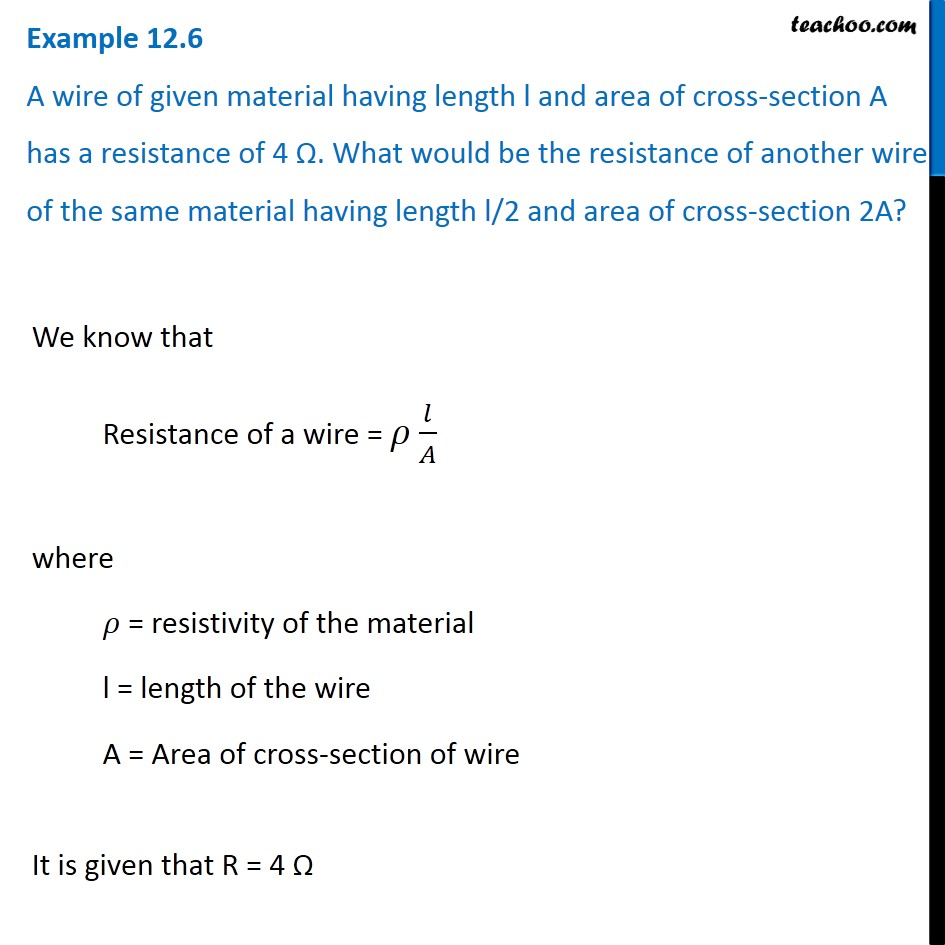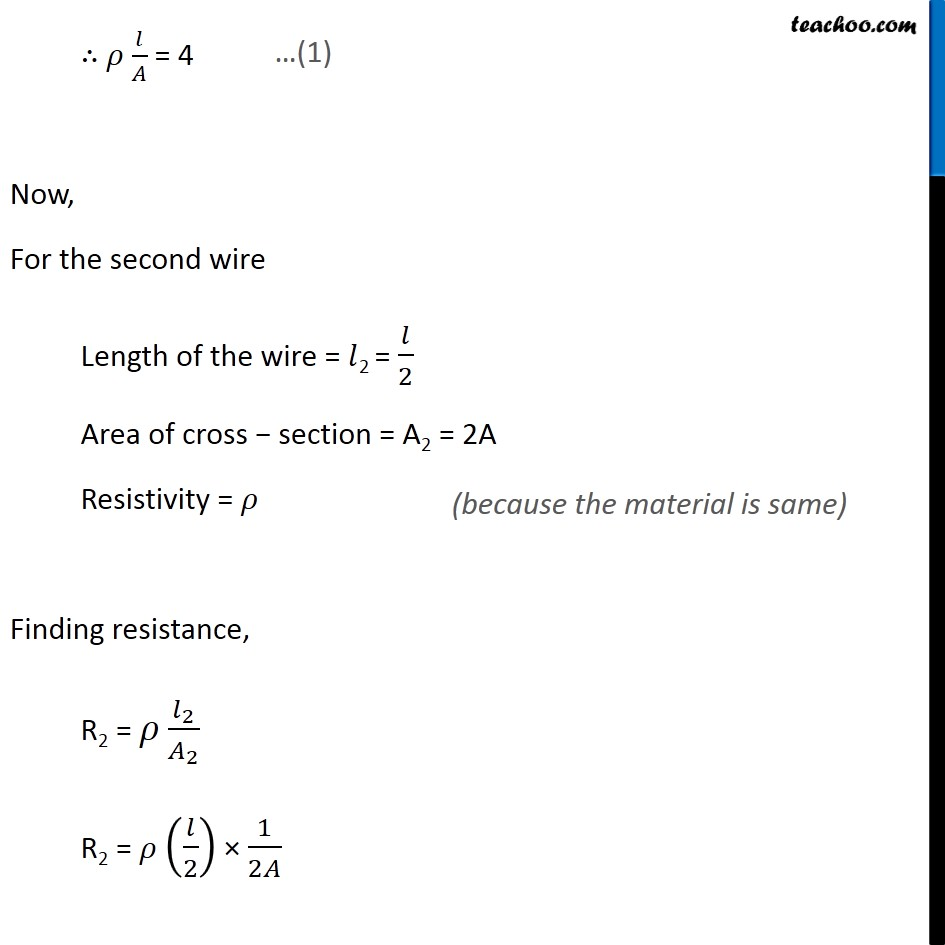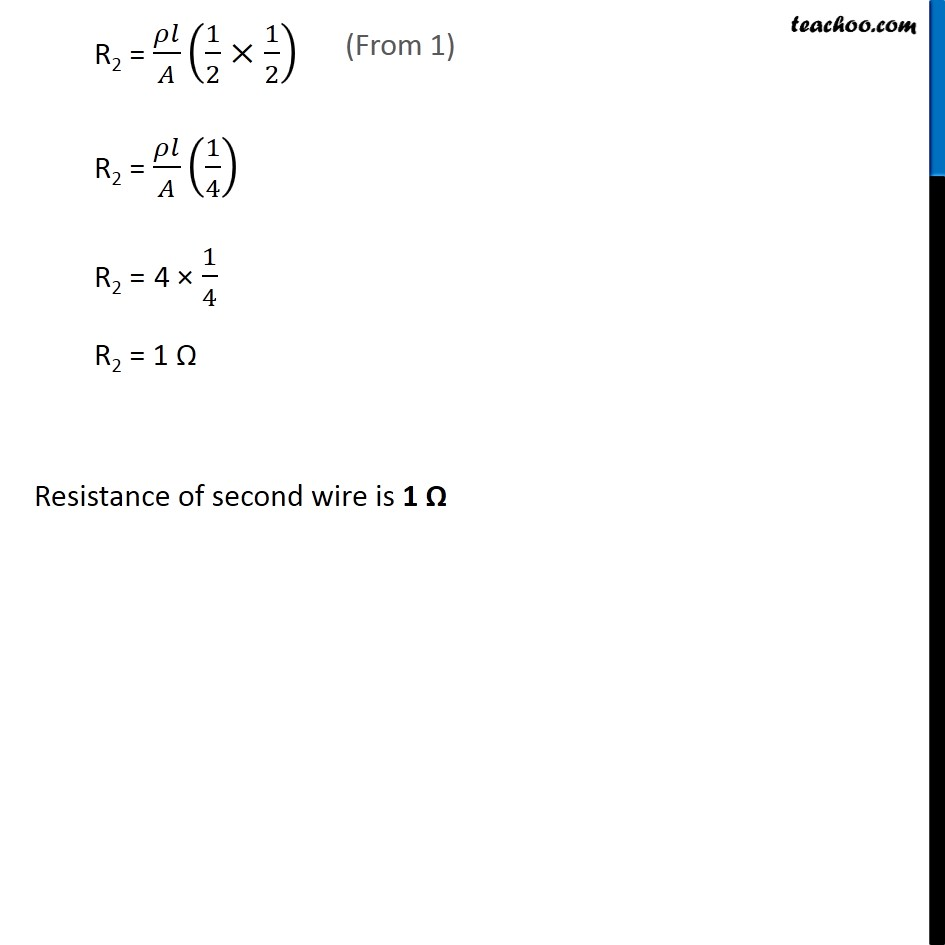Examples from NCERT Book

Class 10
Chapter 12 Class 10 - Electricity

## A wire of given material having length l and area of cross-section A has a resistance of 4 Ω. What would be the resistance of another wire of the same material having length l/2 and area of cross-section 2A?Learn in your speed, with individual attention - Teachoo Maths 1-on-1 Class

### Transcript

Example 12.6 A wire of given material having length l and area of cross-section A has a resistance of 4 Ω. What would be the resistance of another wire of the same material having length l/2 and area of cross-section 2A? We know that Resistance of a wire = 𝜌 𝑙/𝐴 where 𝜌 = resistivity of the material l = length of the wire A = Area of cross-section of wire It is given that R = 4 Ω ∴ 𝜌 𝑙/𝐴 = 4 Now, For the second wire Length of the wire = 𝑙2 = 𝑙/2 Area of cross − section = A2 = 2A Resistivity = 𝜌 Finding resistance, R2 = 𝜌 𝑙_2/𝐴_2 R2 = 𝜌 (𝑙/2) × 1/2𝐴 (because the material is same) R2 = 𝜌𝑙/𝐴 (1/2×1/2) R2 = 𝜌𝑙/𝐴 (1/4) R2 = 4 × 1/4 R2 = 1 Ω Resistance of second wire is 1 Ω